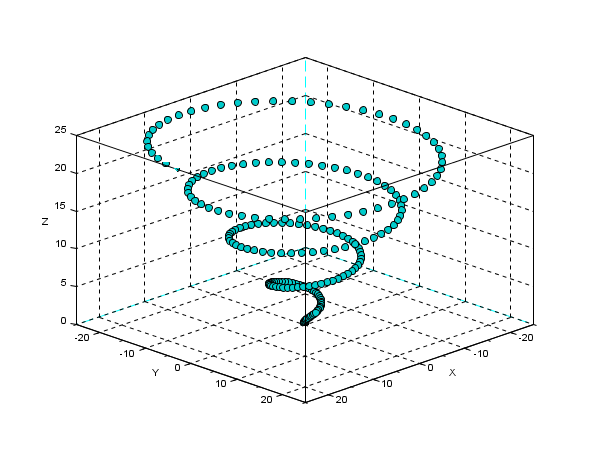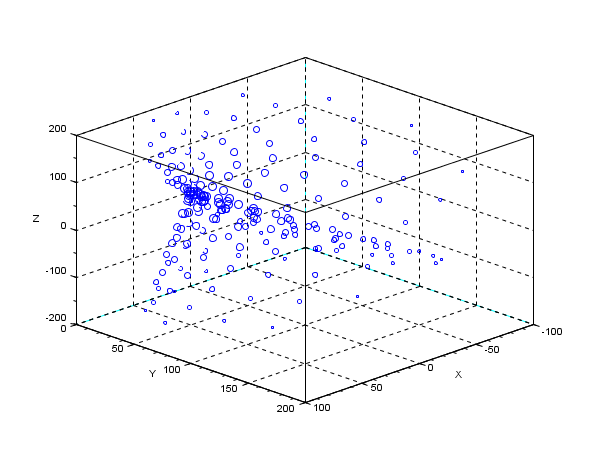Scilab Home page | Wiki | Bug tracker | Forge | Mailing list archives | ATOMS | File exchange
Change language to: English - Français - Português - 日本語 -

Справка Scilab >> Графики > 3d_plot > scatter3

# scatter3

3D scatter plot

### Syntax

```polyline = scatter3(x, y, z, msizes, mcolors, "fill", marker, <marker_property,value>)
polyline = scatter3(axes_handle,...)```

### Arguments

x, y, z

columns or rows vectors of n real numbers specifying the `x`, `y` and `z` coordinates of the centers of markers.

msizes

Specifying the sizes of the markers. To plot each marker with equal size, specify `msizes` as a scalar. To plot each marker with a different size, specify `msizes` as a vector with n components. The units for `msizes` is points squared, with a default value of 36 points squared. The size equals the area of the circle surrounding the marker.

mcolors

Specifying the colors of the markers. To plot each marker with the same color, specify `mcolors` as a string representing a known color: see color_list. A color can also be specified by a vector of 3 RGB values. RGB values correspond to red, green and blue intensity between 0 and 1. To plot each marker with a different color, specify `mcolors` as a vector with n components. The values of `mcolors` are linearly mapped to the colors in the current colormap. Colors can also by specified by a matrix of RGB values or a vector of strings representing known colors. A matrix of RGB values has 3 columns and n rows. The default color is "blue".

"fill"

This string indicates that the markers are filled with some colors. By default the filling colors of markers are the same as their shape ones. However, both colors can be set independently by using the properties `"markerFaceColor"` or `"markerBackgroundColor"`.

marker

Select the type of the marker. The same marker shape is used for all specified points. The figure below shows the 15 different marker types.Marker types are specified by strings: see the table below.

 String Marker type `"."` Point `"+"` Plus sign `"x"` Cross `"circle plus"` Circle with plus `"filled diamond"` Filled diamond `"d"` or `"diamond"` Diamond `"^"` Upward-pointing triangle `"v"` Downward-pointing triangle `"diamond plus"` Diamond with plus `"o"` Circle (default) `"*"` Asterisk `"s"` or `"square"` Square `">"` Right-pointing triangle `"<"` Left-pointing triangle `"pentagram"` or `"p"` Five-pointed star

<marker_property, value>

A sequence of property value pairs can be used to specify type, color and line width of the markers.

<"marker", value> or <"markerStyle", value>

Specify the type of the marker, see the table above.

<"markerEdgeColor", value> or <"markerForeground", value>

Specify the foreground color of the marker. A color can be specified by a string representing a known color: see color_list. A color can also be specified by a vector of 3 RGB values. RGB values correspond to red, green and blue intensity between 0 and 1. This option is superseded by the `mcolors` argument.

<"markerFaceColor", value> or <"markerBackground", value>

Specify the background color of the marker. A color can be specified by a string representing a known color: see color_list. A color can also be specified by a vector of 3 RGB values. RGB values correspond to red, green and blue intensity between 0 and 1. This option is superseded by argument `mcolors`.

<"linewidth", value> or <"thickness", value>

Specify the thickness of the edge of the marker. The unit for the value is one point.

axes_handle

This optional argument forces the scatter plot to appear inside the selected axes given by `axes_handle` rather than inside the current axes, see gca.

polyline

Handle of the created polyline.

### Description

`scatter3(x,y,z)` creates a scatter plot with markers at the locations specified by `x`, `y`, and `z`. The default type of the marker is a circle, the default color is "blue" and the default marker size is 36. This means the circle surrounding the marker has an area of 36 points squared.

Using `scatter3(x,y,z,s,c)` different sizes and colors for each marker can be specified. There are many different ways to specify marker types, marker colors and marker sizes. For more details see the description of the arguments and the examples.To skip an argument, just replace it with `[]` as in `scatter3(x,y,z,[],mcolors)`. When the axes is rotated, the markers are automatically rotated as well in order to show always the same face to the observer.

### Examples

Create 3D scatter plot

```// x, y and z initialisation
t = 1:200;
x = t .* cos(t) .* sin(t);
y = t .* sin(t) .* sin(t);
z = t .* cos(t);
// create 3D scatter plot
scatter3(x, y, z);
// modify rotation angles
gca().rotation_angles = [60, 45];```Vary marker size

```// x, y and z initialisation
t = 1:200;
x = t .* cos(t) .* sin(t);
y = t .* sin(t) .* sin(t);
z = t .* cos(t);
s = linspace(50, 1, length(t));
// create 3D scatter plot
scatter3(x, y, z, s);
// modify rotation angles
gca().rotation_angles = [60, 45];```Vary marker size and color

```// x, y and z initialisation
t = 1:200;
x = t .* cos(t) .* sin(t);
y = t .* sin(t) .* sin(t);
z = t .* cos(t);
s = linspace(50, 1, length(t));
// set color map
gcf().color_map = coolcolormap(64);
c = t;
// create 3D scatter plot
scatter3(x, y, z, s, c);
// modify rotation angles
gca().rotation_angles = [60, 45];```Fill the markers

```// x, y and z initialisation
z = linspace(0, 25, 200);
x = z .* cos(z);
y = z .* sin(z);
// create 3D scatter plot
scatter3(x, y, z, "fill");
// modify rotation angles
gca().rotation_angles = [60, 45];```Specify marker symbol

```// x, y and z initialisation
z = linspace(0, 25, 200);
x = z .* cos(z);
y = z .* sin(z);
// create 3D scatter plot
scatter3(x, y, z, "*");
// modify rotation angles
gca().rotation_angles = [60, 45];```Change marker edge and face color

```// x, y and z initialisation
z = linspace(0, 25, 200);
x = z .* cos(z);
y = z .* sin(z);
// create 3D scatter plot
scatter3(x, y, z,...
"markerEdgeColor", "black",...
"markerFaceColor", [0 .8 .8]);
// modify rotation angles
gca().rotation_angles = [60, 45];```Specify subplot for scatter plot

```// x, y and z initialisation
n = 20;
[x, y] = meshgrid(linspace(-2, 2, n));
z = exp(-x.^2 - y.^2);
// create 3D scatter subplot
subplot(2,1,1)
scatter3(gca(), x(:), y(:), z(:));
// modify rotation angles
gca().rotation_angles = [60,45];
// create 3D scatter subplot
subplot(2,1,2)
scatter3(gca(), x(:), y(:), z(:), "markerFaceColor", [0 .8 .8]);
// modify rotation angles
gca().rotation_angles = [60,45];```Modify scatter plot after creation

```// x, y and z initialisation
t = 1:200;
x = t .* cos(t) .* sin(t);
y = t .* sin(t) .* sin(t);
z = t .* cos(t);
s = linspace(50, 1, length(t));
// create 3D scatter plot
scatter3(x, y, z, s);
// modify rotation angles
gca().rotation_angles = [60,45];``````// modify polyline
p.mark_foreground = color(0.5, 0, 0);
p.mark_background = color(0.5, 0.5, 0);```• scatter — 2D scatter plot
• param3d — plots a single curve in a 3D cartesian frame
• gca — Return handle of current axes.
• gcf — Return handle of current graphic window.
• color_list — list of named colors
• polyline_properties — description of the Polyline entity properties

### History

 Version Description 6.0.0 Function `scatter3` introduced.